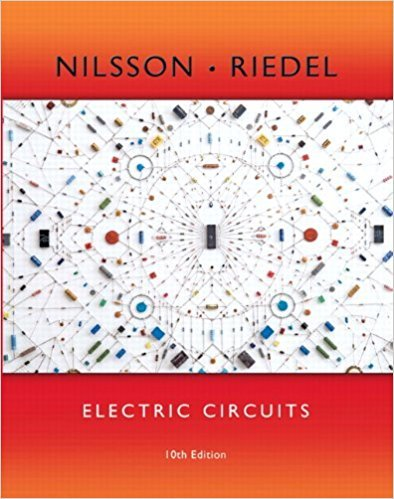×
×

# Solved: a) Use a series of source transformations to find in the circuit in Fig. P4.62ISBN: 9780133760033 390

## Solution for problem 4.62 Chapter 4

Electric Circuits | 10th Edition

• Textbook Solutions
• 2901 Step-by-step solutions solved by professors and subject experts
• Get 24/7 help from StudySoup virtual teaching assistantsElectric Circuits | 10th Edition

4 5 1 285 Reviews
10
0
Problem 4.62

a) Use a series of source transformations to find in the circuit in Fig. P4.62. b) Verify your solution by using the mesh-current method to find io

Step-by-Step Solution:
Step 1 of 3

l,ACrt (x()= =7f Ynr+ - r- tP) E : Y, + -.: l',Oz pt)T .;h J\. VorCu O ig.^) +N .-\z =- t 14'l, ,ltr 41 /...

Step 2 of 3

Step 3 of 3

##### ISBN: 9780133760033

The answer to “a) Use a series of source transformations to find in the circuit in Fig. P4.62. b) Verify your solution by using the mesh-current method to find io” is broken down into a number of easy to follow steps, and 27 words. Since the solution to 4.62 from 4 chapter was answered, more than 214 students have viewed the full step-by-step answer. The full step-by-step solution to problem: 4.62 from chapter: 4 was answered by , our top Engineering and Tech solution expert on 03/13/18, 07:48PM. This textbook survival guide was created for the textbook: Electric Circuits, edition: 10. Electric Circuits was written by and is associated to the ISBN: 9780133760033. This full solution covers the following key subjects: . This expansive textbook survival guide covers 107 chapters, and 1357 solutions.

Unlock Textbook Solution